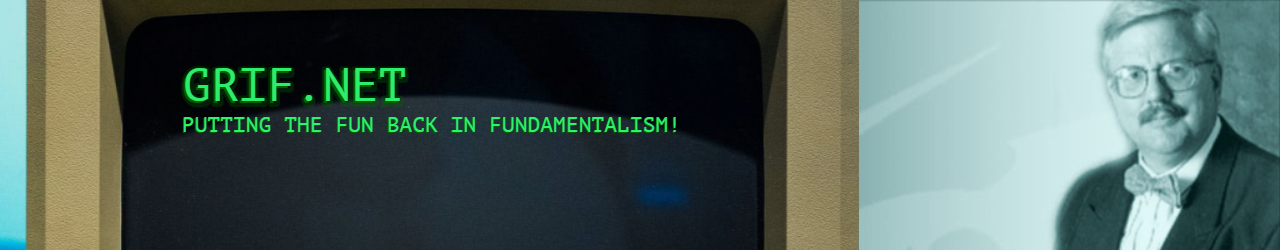# 08/05/16 Grif.Net – Talking with Vets

## 08/05/16 Grif.Net – Talking with Vets

[Real stories of =
interviews with our veterans]

My high school assignment =E2=80=A8was to ask a veteran =
about World War II. Since my father had served =E2=80=A8in the Philippines =
during the war, I chose him. After a few basic questions, I very =
gingerly asked, =E2=80=9CDid you ever kill =
anyone?=E2=80=9D

Dad got quiet. Then, in a soft voice, he =
said, =E2=80=9CProbably. I was the cook.=E2=80=9D

~~
My wife at the VA =
hospital, entered a patient=E2=80=99s room to draw blood. Noticing an =
apple on his nightstand, she remarked, =E2=80=9CAn apple a day keeps the =
doctor away, right?=E2=80=9D

=E2=80=9CThat must be true,=E2=80=9D he =
agreed. =E2=80=9CI haven=E2=80=99t seen a doctor in three =
days.=E2=80=9D

~~
period on his World War II experiences, someone asked, =E2=80=9CHow did =
you know the war was over?=E2=80=9D

He replied, =E2=80=9CWhen everyone stopped =
shooting at me.=E2=80=9D

~~
After a parishioner returned from =
her second tour in Iraq, I remarked on her beautiful complexion. =
=E2=80=9CWhat do you use on your face out there in the desert to keep it =
so smooth?=E2=80=9D.

=E2=80=9CNothing,=E2=80=9D she replied. =
=E2=80=9CIt gets sandblasted every day.=E2=80=9D

~~

Dr Bob Griffin =

bob@grif.net www.grif.net =

"Jesus Knows Me, This I =
Love!"